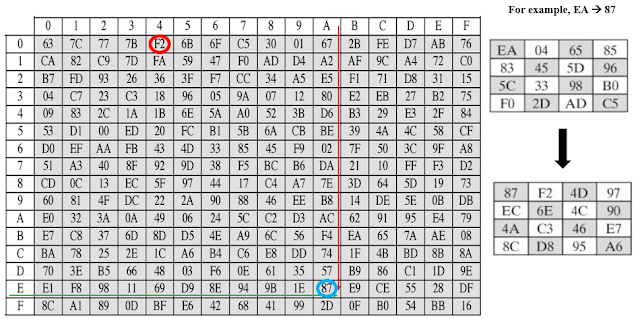## Wednesday, September 8, 2021

### AES Algorithm | Advance Encryption Standard Algorithm | AES Encryption Process

Introduction

AES is symmetric key cryptographic algorithm published by NIST. The algorithm was proposed by Rijndael. It is also known as Rijndael encryption algorithm. AES is replacement of DES. AES works on block cipher technique. Size of plain text and cipher text must be same. An input key is also required input to the AES algorithm. Same size of plain text.

In AES, the data length (plain text size) of 128 bits, and supporting three different key lengths, 128, 192 and 256 bits. AES consists of multiple rounds of processing different key bits like 10 rounds for processing 128-bit keys, 12 rounds for processing 192-bit keys, and 14 rounds for processing 256-bit keys.

Plain Text transform in Matrix Form

Plain text (128-bit) converts into 4x4 matrix of bytes. Therefore, the first four bytes of a 128-bit input block occupy first column in the 4x4 matrix of bytes. The next four bytes occupy the second column, and so on. AES operates on a 4x4 column-major order matrix of bytes; called as state array.

For Example, “AES USES A MATRIX”.

Steps of AES Encryption

Overall structure of AES encryption process shown in figure. The number of rounds is 10, for the case when the encryption key is 128 bit long. (12 rounds – 192 bits, 14-rounds – 256 bits).

For encryption, each round consists of the following four steps: SubBytes, ShiftRows, MixColumns, AddRoundKey

Above steps also called AES transformation function.Figure : AES Encryption Process Steps

SubBytes / Substitute Bytes

AES defines a 16 x 16 matrix of byte values, called an S-box, that contains a permutation of all possible 256 8-bit values.  Each individual byte of State is mapped into a new byte in the following way: The leftmost 4 bits of the byte are used as a row value and the rightmost 4 bits are used as a column value. These row and column values serve as indexes into the S-box to select a unique 8-bit output value.

For example, the hexadecimal value {EA} references row E, column A of the S-box, which contains the value {87}. Accordingly, the value {EA} is mapped into the value {87}.

Below table is S-box and used during encryption process.Figure : Substituion Box with Example

Below table is inverse S-box. It will be used during decryption process.Figure : Inverse S - box with Example

Shift Row transformation

The shift row transformation is called ShiftRows.

Rules of shifting rows,

Row 1  No Shifting

Row 2  1 byte left shift

Row 3  2 byte left shift

Row 4  3 byte left shift

The inverse shift row transformation, called InvShiftRows, performs the circular shifts in the opposite direction for each of the last three rows, with a one-byte circular right shift for the second row, and so on.Figure : Shift Rows and Inverse Shift Rows with Example

Mix Columns

The mix column transformation, called MixColumns, operates on each column individually. Each byte of a column is mapped into a new value that is a function of all four bytes in that column. The mix column transformation, called MixColumns, operates on each column individually. Each byte of a column is mapped into a new value that is a function of all four bytes in that column.

In the forward add round key transformation, called AddRoundKey, the 128 bits of State are bitwise XORed with the 128 bits of the round key.  As shown in figure, the operation is viewed as a column wise operation between the 4 bytes of a state column and one word of the round key; it can also be viewed as a byte-level operation.